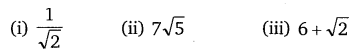# CLASS 10 MATH NCERT SOLUTIONS FOR CHAPTER – 1 REAL NUMBERS EX – 1.3

## Real Numbers

Question 1.
Prove that √5 is irrational.
Solution:
Let √5 =$\frac { p }{ q }$ be a rational number, where p and q are co-primes and q ≠ 0.
Then, √5q = p => 5q2=p2
⇒  p2 – Sq2     … (i)
Since 5 divides p2, so it will divide p also.
Let p = 5r
Then p2 – 25r 2     [Squaring both sides]
⇒ 5q2 = 25r2     [From(i)]
⇒ q2 = 5r2
Since 5 divides q2, so it will divide q also. Thus, 5 is a common factor of both p and q.
This contradicts our assumption that √5 is rational.
Hence, √5 is irrational. Hence, proved.

Question 2.
Show that 3 + √5 is irrational.

Solution:
Let 3 + 2√5 =$\frac { p }{ q }$ be a rational number, where p and q are co-prime and q ≠ 0.
Then, 2√5 =$\frac { p }{ q }$ – 3 =$\frac { p - 3q }{ q }$
⇒ √5 =$\frac { p - 3q }{ 2q }$
since$\frac { p - 3q }{ 2q }$ is a rational number,
therefore, √5 is a rational number. But, it is a contradiction.
Hence, 3 + √5 is irrational. Hence, proved.

Question 3.
Prove that the following are irrational.Solution:
(i) Let$\frac { 1 }{ \sqrt { 2 } }$ =$\frac { p }{ q }$ be a rational number,
where p and q are co-prime and q ≠ 0.
Then, √2 =$\frac { q }{ p }$
Since$\frac { q }{ p }$ is rational, therefore, √2 is rational.
But, it is a contradiction that √2 is rational, rather it is irrational.
Hence,$\frac { 1 }{ \sqrt { 2 } }$ is irrational.
Hence, proved.

(ii) Let 7√5 =$\frac { p }{ q }$ be a rational number, where p, q are co-primes and q ≠ 0.
Then, √5 =$\frac { p }{ 7q }$
Since$\frac { p }{ 7q }$ is rational therefore, √5 is rational.
But, it is a contradiction that √5 is rational rather it is irrational.
Hence, 7√5 s is irrational.
Hence proved.

(iii) Let 6 + √2 =$\frac { p }{ q }$ be a rational number, where p, q are co-primes and q ≠ 0.
Then, √2 =$\frac { p }{ q }$ – 6 =$\frac { p - 6q }{ q }$
Since$\frac { p - 6q }{ q }$ is rational therefore, √2 is rational.
But, it is a contradiction that √2 is rational, rather it is irrational.
Hence, 6 + √2 is irrational.

#### CLASS 10 MATH NCERT SOLUTIONS FOR CHAPTER – 1 REAL NUMBERS EX – 1.4

Lorem ipsum dolor sit amet, consectetur adipiscing elit. Phasellus cursus rutrum est nec suscipit. Ut et ultrices nisi. Vivamus id nisl ligula. Nulla sed iaculis ipsum.

Company Name